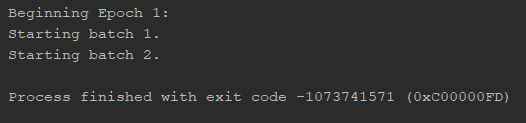# LSTM with long inputs halts with no error message

Hello,

I’m having issues training a very basic LSTM with inputs of 5000 time steps. It always halts on the second mini-batch during the loss calculation on a weird error code, and there is no error message:I’ve tried changing the input to all zeros, changing the optimizer, changing the loss function, etc. None of these fixed the issue. The problem also happens when I replace the LSTM with an RNN or LSTMCell. If I remove the mini-batches and train with full-batch instead, it still breaks on the second epoch.

Strangely, it works if I reduce my input time steps to lower numbers. Specifically, an input length of 1046 time steps will work, but 1047 will not.

I’m not even using a GPU yet, but of course the problem persists when I do.

Here is my code:

``````class Test_LSTM(torch.nn.Module):

def __init__(self, input_dim, hidden_dim, batch_size, output_dim=1,
num_layers=1, use_cuda=False):
super(Test_LSTM, self).__init__()
self.input_dim = input_dim
self.hidden_dim = hidden_dim
self.batch_size = batch_size
self.num_layers = num_layers
self.use_cuda = use_cuda

self.lstm = torch.nn.LSTM(self.input_dim, self.hidden_dim, self.num_layers, batch_first=True)
self.linear = torch.nn.Linear(self.hidden_dim, output_dim)
self.sigmoid = torch.nn.Sigmoid()

def init_hidden(self):
if self.use_cuda:
return (Variable(torch.zeros(self.num_layers, self.batch_size, self.hidden_dim).cuda()),
Variable(torch.zeros(self.num_layers, self.batch_size, self.hidden_dim).cuda()))
return (Variable(torch.zeros(self.num_layers, self.batch_size, self.hidden_dim)),
Variable(torch.zeros(self.num_layers, self.batch_size, self.hidden_dim)))

def forward(self, input):
lstm_out, self.hidden = self.lstm(input, self.hidden)
y_pred = self.sigmoid(self.linear(lstm_out[:, -1, :]))
return y_pred

def create_loss_and_optimizer(self, learning_rate=0.001):
loss = torch.nn.BCELoss()

return loss, optimizer

def fit(self, X, y, num_epochs=20, learning_rate=0.001, verbose=False):
criterion, optimizer = self.create_loss_and_optimizer(learning_rate=learning_rate)

for epoch in range(num_epochs):
print("\nBeginning Epoch {}:".format(epoch + 1))

for i in range((len(X) - 1) // self.batch_size + 1):
print("Starting batch {}.".format(i + 1))
self.hidden = self.init_hidden()

# Convert torch tensor to Variable
if i * self.batch_size + self.batch_size < len(X):
X_var = Variable(X[i * self.batch_size: (i + 1) * self.batch_size])
y_var = Variable(y[i * self.batch_size: (i + 1) * self.batch_size])
else:
X_var = Variable(X[i * self.batch_size:])
y_var = Variable(y[i * self.batch_size:])

# Forward + Backward + Optimize